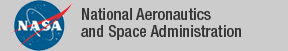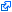### Interval Arithmetic

The PVSdevelopment `interval_arith`, which is part of the NASA PVS Library, formalizes the theory of interval arithmetic for a large set of expressions including special real-valued function such as square root, trigonometric functions, and logarithm and exponential functions. The development includes proof-producing stragegies for reasoning about numerical expressions.

### Strategies

The following strategies are implemented via computational reflection using a verified generic branch and bound algorithm (see `structures/branch_and_bound.pvs`).

• `numerical`: Estimate the minimum and maximum values of real-valued expressions. The accuracy of the estimation can be specified by the user.
• `interval`: Check validity and satisfiability of relational formulas of real-valued expressions.
• Examples: rational expressions, real-valued expressions.

### Publications

The tagidentifies links that are outside the NASA domain